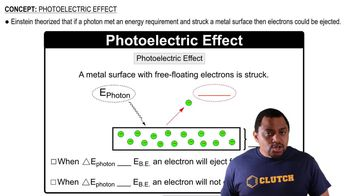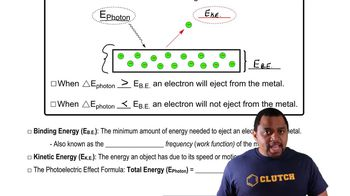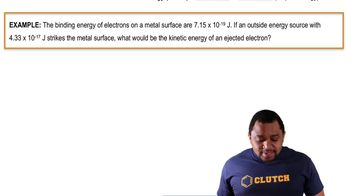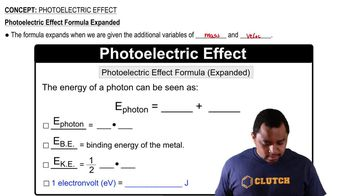Start typing, then use the up and down arrows to select an option from the list.# Photoelectric Effect

Jules Bruno
220views
1
now the photo electric effect formula can be expanded when we're given the additional variables of mass and velocity. So here we're going to say with the new expanded formula that the energy of a photon can be seen as energy of a photon equals your binding energy plus your kinetic energy. And when it comes to buying the energy of a photon, we're gonna say it's equal to Planck's constant, which is H. Times your frequency which is mu binding energy. Remember is just abbreviated as B. E. And your kinetic energy energy of motion equals a half times the mass of the electron. In this case times velocity squared. Now sometimes you'll see instead of jewels or killer jewels being used, we might see E. V. Which stands for electron volts. So just remember here that one electron volt equals 1.602 times 10 to the negative 19 jewels. So just remember if energy is given in electron volts, then use the conversion factor to change it into jewels. Okay, so with our new expanded formula, we could substitute this in for the energy of a photon and we could substitute this in for the kinetic energy of the ejected electron01:2601:1101:2201:1801:52